# WO2010064396A1 - Moving picture decoding method and moving picture encoding method - Google Patents

Moving picture decoding method and moving picture encoding method Download PDF

## Info

Publication number
WO2010064396A1
WO2010064396A1 PCT/JP2009/006438 JP2009006438W WO2010064396A1 WO 2010064396 A1 WO2010064396 A1 WO 2010064396A1 JP 2009006438 W JP2009006438 W JP 2009006438W WO 2010064396 A1 WO2010064396 A1 WO 2010064396A1
Authority
WO
WIPO (PCT)
Prior art keywords
motion vector
vector
prediction
block
motion
Prior art date
Application number
PCT/JP2009/006438
Other languages
French (fr)
Japanese (ja)
Inventor

Original Assignee

Priority date (The priority date is an assumption and is not a legal conclusion. Google has not performed a legal analysis and makes no representation as to the accuracy of the date listed.)
Filing date
Publication date
Priority to JP2008308113 priority Critical
Priority to JP2008-308113 priority
Application filed by 株式会社日立製作所 filed Critical 株式会社日立製作所
Publication of WO2010064396A1 publication Critical patent/WO2010064396A1/en

## Images

•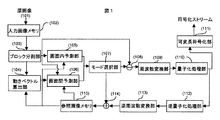•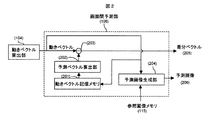•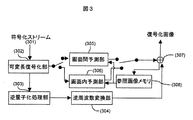•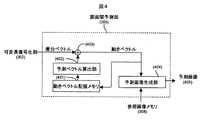•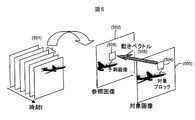•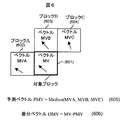•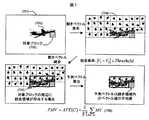•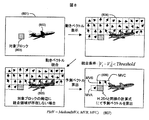•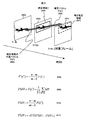•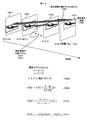•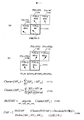•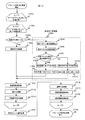•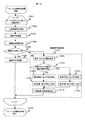•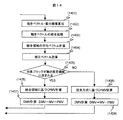•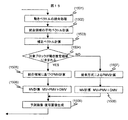•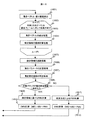•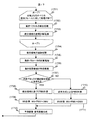•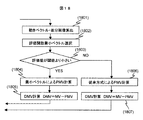•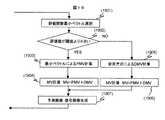## Classifications

• HELECTRICITY
• H04ELECTRIC COMMUNICATION TECHNIQUE
• H04NPICTORIAL COMMUNICATION, e.g. TELEVISION
• H04N19/00Methods or arrangements for coding, decoding, compressing or decompressing digital video signals
• H04N19/50Methods or arrangements for coding, decoding, compressing or decompressing digital video signals using predictive coding
• H04N19/503Methods or arrangements for coding, decoding, compressing or decompressing digital video signals using predictive coding involving temporal prediction
• H04N19/51Motion estimation or motion compensation
• H04N19/513Processing of motion vectors
• H04N19/517Processing of motion vectors by encoding
• H04N19/52Processing of motion vectors by encoding by predictive encoding
• HELECTRICITY
• H04ELECTRIC COMMUNICATION TECHNIQUE
• H04NPICTORIAL COMMUNICATION, e.g. TELEVISION
• H04N19/00Methods or arrangements for coding, decoding, compressing or decompressing digital video signals
• H04N19/10Methods or arrangements for coding, decoding, compressing or decompressing digital video signals using adaptive coding
• H04N19/102Methods or arrangements for coding, decoding, compressing or decompressing digital video signals using adaptive coding characterised by the element, parameter or selection affected or controlled by the adaptive coding
• H04N19/124Quantisation
• HELECTRICITY
• H04ELECTRIC COMMUNICATION TECHNIQUE
• H04NPICTORIAL COMMUNICATION, e.g. TELEVISION
• H04N19/00Methods or arrangements for coding, decoding, compressing or decompressing digital video signals
• H04N19/10Methods or arrangements for coding, decoding, compressing or decompressing digital video signals using adaptive coding
• H04N19/102Methods or arrangements for coding, decoding, compressing or decompressing digital video signals using adaptive coding characterised by the element, parameter or selection affected or controlled by the adaptive coding
• H04N19/13Adaptive entropy coding, e.g. adaptive variable length coding [AVLC] or context adaptive binary arithmetic coding [CABAC]
• HELECTRICITY
• H04ELECTRIC COMMUNICATION TECHNIQUE
• H04NPICTORIAL COMMUNICATION, e.g. TELEVISION
• H04N19/00Methods or arrangements for coding, decoding, compressing or decompressing digital video signals
• H04N19/10Methods or arrangements for coding, decoding, compressing or decompressing digital video signals using adaptive coding
• H04N19/134Methods or arrangements for coding, decoding, compressing or decompressing digital video signals using adaptive coding characterised by the element, parameter or criterion affecting or controlling the adaptive coding
• H04N19/157Assigned coding mode, i.e. the coding mode being predefined or preselected to be further used for selection of another element or parameter
• H04N19/159Prediction type, e.g. intra-frame, inter-frame or bidirectional frame prediction
• HELECTRICITY
• H04ELECTRIC COMMUNICATION TECHNIQUE
• H04NPICTORIAL COMMUNICATION, e.g. TELEVISION
• H04N19/00Methods or arrangements for coding, decoding, compressing or decompressing digital video signals
• H04N19/44Decoders specially adapted therefor, e.g. video decoders which are asymmetric with respect to the encoder
• HELECTRICITY
• H04ELECTRIC COMMUNICATION TECHNIQUE
• H04NPICTORIAL COMMUNICATION, e.g. TELEVISION
• H04N19/00Methods or arrangements for coding, decoding, compressing or decompressing digital video signals
• H04N19/50Methods or arrangements for coding, decoding, compressing or decompressing digital video signals using predictive coding
• H04N19/503Methods or arrangements for coding, decoding, compressing or decompressing digital video signals using predictive coding involving temporal prediction
• H04N19/51Motion estimation or motion compensation
• HELECTRICITY
• H04ELECTRIC COMMUNICATION TECHNIQUE
• H04NPICTORIAL COMMUNICATION, e.g. TELEVISION
• H04N19/00Methods or arrangements for coding, decoding, compressing or decompressing digital video signals
• H04N19/60Methods or arrangements for coding, decoding, compressing or decompressing digital video signals using transform coding
• H04N19/61Methods or arrangements for coding, decoding, compressing or decompressing digital video signals using transform coding in combination with predictive coding
• HELECTRICITY
• H04ELECTRIC COMMUNICATION TECHNIQUE
• H04NPICTORIAL COMMUNICATION, e.g. TELEVISION
• H04N19/00Methods or arrangements for coding, decoding, compressing or decompressing digital video signals
• H04N19/10Methods or arrangements for coding, decoding, compressing or decompressing digital video signals using adaptive coding
• H04N19/169Methods or arrangements for coding, decoding, compressing or decompressing digital video signals using adaptive coding characterised by the coding unit, i.e. the structural portion or semantic portion of the video signal being the object or the subject of the adaptive coding
• H04N19/17Methods or arrangements for coding, decoding, compressing or decompressing digital video signals using adaptive coding characterised by the coding unit, i.e. the structural portion or semantic portion of the video signal being the object or the subject of the adaptive coding the unit being an image region, e.g. an object
• H04N19/172Methods or arrangements for coding, decoding, compressing or decompressing digital video signals using adaptive coding characterised by the coding unit, i.e. the structural portion or semantic portion of the video signal being the object or the subject of the adaptive coding the unit being an image region, e.g. an object the region being a picture, frame or field
• HELECTRICITY
• H04ELECTRIC COMMUNICATION TECHNIQUE
• H04NPICTORIAL COMMUNICATION, e.g. TELEVISION
• H04N19/00Methods or arrangements for coding, decoding, compressing or decompressing digital video signals
• H04N19/10Methods or arrangements for coding, decoding, compressing or decompressing digital video signals using adaptive coding
• H04N19/169Methods or arrangements for coding, decoding, compressing or decompressing digital video signals using adaptive coding characterised by the coding unit, i.e. the structural portion or semantic portion of the video signal being the object or the subject of the adaptive coding
• H04N19/17Methods or arrangements for coding, decoding, compressing or decompressing digital video signals using adaptive coding characterised by the coding unit, i.e. the structural portion or semantic portion of the video signal being the object or the subject of the adaptive coding the unit being an image region, e.g. an object
• H04N19/176Methods or arrangements for coding, decoding, compressing or decompressing digital video signals using adaptive coding characterised by the coding unit, i.e. the structural portion or semantic portion of the video signal being the object or the subject of the adaptive coding the unit being an image region, e.g. an object the region being a block, e.g. a macroblock
• HELECTRICITY
• H04ELECTRIC COMMUNICATION TECHNIQUE
• H04NPICTORIAL COMMUNICATION, e.g. TELEVISION
• H04N19/00Methods or arrangements for coding, decoding, compressing or decompressing digital video signals
• H04N19/10Methods or arrangements for coding, decoding, compressing or decompressing digital video signals using adaptive coding
• H04N19/169Methods or arrangements for coding, decoding, compressing or decompressing digital video signals using adaptive coding characterised by the coding unit, i.e. the structural portion or semantic portion of the video signal being the object or the subject of the adaptive coding
• H04N19/184Methods or arrangements for coding, decoding, compressing or decompressing digital video signals using adaptive coding characterised by the coding unit, i.e. the structural portion or semantic portion of the video signal being the object or the subject of the adaptive coding the unit being bits, e.g. of the compressed video stream

## Abstract

High-quality video is provided using a small amount of coding. The moving picture decoding method performs inter-frame prediction processing. With the aforementioned inter-frame prediction processing, blocks with similar motion vectors from among the motion vectors in multiple blocks that have already been decoded are consolidated and a consolidated area is computed. A predicted vector for a target block to be decoded is computed using the motion vector of the aforementioned consolidated area, and a motion vector for the aforementioned target block is computed based on the aforementioned predicted vector and a difference vector which is included in a coded stream that is input. A predicted image is generated using the aforementioned motion vector, and a difference image which is included in the aforementioned coded stream and the aforementioned predicted image are added to generate a decoded image.

## Description

Moving picture decoding method and the moving picture coding method

The present invention relates to moving picture encoding technique for encoding the moving picture decoding technique and the moving picture decoding a moving image.

By using the image information encoding processing has been completed to predict the encoding target image block by block, by coding the prediction difference between the original image and reduce the amount of codes except redundancy possessed by the moving image technology There has been disclosed. However, it is necessary to encode the motion vector result of block search in addition to the predicted difference, the code amount of overhead.

In H.264 / AVC (Non-Patent Document 1), in order to reduce the code amount of the motion vector, discloses a prediction technique for the motion vector. That is, when coding a motion vector to predict the motion vector of the target block using the coded blocks located around the target block, the variable difference (difference vector) of the prediction vector and the motion vector to length encoding. However, it can not be said that the prediction accuracy of a motion vector is sufficient, such as in particular moving objects there are a plurality, there is a problem that it requires many code amount still motion vector for the motion of complex image .

ITU-T Recommendation H.264 / AVC

The above technique, since the prediction accuracy of the motion vector is not sufficiently high, it is still necessary number of code amount to the motion vector.

The present invention has been made in view of the above problems, its object is to reduce the code amount of motion vectors to improve the method of calculating the predicted vector, and to improve the compression efficiency.

In order to solve the above problems, an embodiment of the present invention, for example, may be configured as described in the appended claims.

It is possible to provide a high quality image with a small amount of code.

Block diagram of an image coding apparatus according to the present invention Block diagram of an image coding apparatus according to the present invention Block diagram of an image decoding apparatus according to the present invention Block diagram of an image decoding apparatus according to the present invention Conceptual illustration of the inter prediction H.264 / AVC video Conceptual illustration Prediction of the motion vectors H.264 / AVC video Conceptual illustration Prediction of the motion vectors in Examples 1, 2 and 3 Conceptual illustration Prediction of the motion vectors in Examples 1, 2 and 3 Conceptual illustration Prediction of the motion vector in Example 2 Conceptual illustration Prediction of the motion vector in Example 3 Conceptual illustration Prediction of the motion vectors in Example 4 Flow diagram of the picture coding method in the present invention Flow diagram of an image decoding method in the present invention Flow diagram of the picture coding method in the present invention Flow diagram of an image decoding method in the present invention Flow diagram of the picture coding method in the present invention Flow diagram of an image decoding method in the present invention Flow diagram of the picture coding method in the present invention Flow diagram of an image decoding method in the present invention

Hereinafter, an embodiment of the present invention will be described with reference to the drawings.

Figure 5 conceptually shows the operation of the inter prediction process by the H.264 / AVC.

In H.264 / AVC, performs coding by blocks in order of raster scanning with respect to the encoding target image. When performing inter prediction, the encoding target image (503) and the reference image to the decoded image of an encoded image contained in the same video (501) (502), the target block in the target image (504) highly correlated block searches from (predicted image) reference in image (505). At this time, in addition to prediction difference is calculated as the difference of both blocks is encoded as a vector (506) moves the difference between the coordinate values ​​of the two blocks. Whereas during decoding may be performed above reverse procedure, by adding the predicted differential decoded block in the reference picture (predictive picture) (505) can acquire the decoded image.

In H.264 / AVC, in order to reduce the code amount of overhead due to motion vectors described above we have introduced prediction technique for the motion vector. That is, when coding a motion vector to predict the motion vector of the target block using the coded blocks located around the target block, a difference (differential vector) between the predicted vector and the motion vector to encode. At this time, since the magnitude of the difference vector is concentrated almost to zero, thereby reducing the amount of code by variable length coding it.

6 conceptually shows a method for calculating the prediction vector. Left of the target block (601), the upper, respectively block A (602) the encoded blocks adjacent to the upper right side, the block B (603), the block and C (604), the motion vector MV of each block MVA, MVB, and MVC. In this case, the predicted vector is MVA, MVB, expressed as the median of MVC. That is, the prediction motion vector PMV, using function Median returns the median value for each component of the specified vector as an argument, is calculated as (605). Furthermore, calculating a difference vector DMV as a difference (606) of the target block and the motion vector MV prediction vector PMV, followed variable length coding the difference vector DMV to. During decoding may be performed above reverse procedure, by adding the decoded difference vector DMV to the prediction motion vector PMV calculated by a procedure similar to that described above, decoding the motion vector MV.

As described above, in H.264 / AVC, by introducing prediction technique for the motion vector, it has become possible to significantly reduce the amount of code required for motion vectors. However, if by H.264 / AVC, only neighboring blocks in the screen when calculating the prediction vector not considered, not necessarily made up it reflects the movement of the object. Therefore, if an object, especially moving in the vicinity area there are a plurality, not sufficient prediction accuracy of a motion vector, it was still requires more code amount to the motion vector. In the present invention, to estimate the presence and range of motion area by integrating those out of the motion vector of the already encoded region is short, the prediction vector based on the motion vector of the moving region existing in the vicinity of the target region by calculating the, it becomes possible to improve the prediction accuracy for the motion vector.

Hereinafter, we describe a method of calculating the prediction vector PMV according to the present invention.

Procedure for calculating the prediction vector PMV is the same on the encoding side and the decoding side, the process of encoding this by calculating a difference vector DMV to a predictive motion vector PMV as motion vector MV in the encoding side is performed. The decoding side is processing for decoding the motion vector MV by adding the prediction vector PMV to the difference vector DMV decoded is performed.

Example 1, when calculating the prediction vector of the target block, using the information of the integration area. That is, if there is a combined area around the target block, and determined to contain part of the same object as the object is also the current block included in the integrated region, the prediction based on the motion vector of a block included in the integrated region vector It is calculated.

Accordingly, it is possible to calculate the prediction vector based on the motion vector of the moving region exists near the target area, it can be improved prediction accuracy for the motion vector. Further, to reduce the code amount of motion vectors to improve the method of calculating the predicted vector can improve the compression efficiency.

Figure 7 is a diagram conceptually showing an example of a prediction motion vector PMV calculation method in the present embodiment.

For example, in the frame (701) to the object (702) is reflected, consider the case of inter-picture coding a block (703) including a part of the object (702). In this case, the motion vector MV (704) in the pre-coding region is first positioned to the left and above the target block (703) and integrated with each other near the motion vector MV between. For example, integrated condition | V1-V2 | if <Threshold is met, by integrating the two motion vectors V1 V2, included in the same area.

Here, | A | a vector A size, and the Threshold constant. Constant Threshold in advance may be set to a unique value, it may be included in the coded stream by freely set the value in the encoding side. Moreover, and encoding information such as the variance value or the average value of the motion vector MV, it may be dynamically determined based on the size, etc. of the integrated region. In addition, it may not be particularly conform to this formula. Furthermore, during the integration, information other than motion vector MV, color information may be considered.

Generally, since the motion vector MV of the region containing the portion of the same object has a property that indicates the values ​​close to each other, able to identify the presence and range of the object (702) by obtaining a combined area (705) it can.

In the present invention, when calculating a prediction motion vector PMV of the target block (703) utilizes information in the combined area (705). That is, if there is a combined area (705) around the target block (703) is the target block (703) is also determined to contain part of the same object as the object (702) contained in the combined area (705) to calculate the prediction vector PMV on the basis of the block motion vectors MV included in the combined area (705). Although not limited the method for calculating the prediction vector PMV in particular, for example, as in Equation 7 (706), may be calculated as the average value of the motion vector MV in the integrated area (AVE (C)), the combined area such as an intermediate value of the motion vector MV of the inner may calculate the prediction vector PMV by selecting a representative motion vector MV in the integrated region.

Here, the set of motion vectors MV included a C to the integration area, | C | is referred to as the number.

Next, the case where there is no combined area (705) around the target block (703). If combined area around the target block (703) (705) is not present, to calculate the prediction vector PMV according to the procedure shown in FIG. 8, for example. Here, in the frame (801) in which an object similar to that of FIG. 7 (802) is reflected, consider the case of inter-picture coding a block (803) away from the object (802). In this case, perform integration by the same procedure as in FIG. 7 with respect to the motion vector MV of the already encoded region (804), obtaining a combined area (805). Then, H.264, etc., to calculate the prediction vector PMV by conventional calculations that use the motion vector MV of the neighboring blocks of the current block (803) (807) (806). However, as the conventional method not limited to H.264.

Next, configuration of a video encoding apparatus according to this embodiment, the operation will be described.

Figure 1 shows an example of a video encoding apparatus according to this embodiment.

Block moving picture coding apparatus in this embodiment, which divides the input image memory for holding inputted original image (101) (102), the image data input from the input image memory (102) into a plurality of regions dividing unit (103), the intra prediction unit which performs intra prediction on a block basis (105), the motion vector calculation unit for performing a motion vector calculated for the image data input from the block division unit (103) (104 a), inter-picture prediction unit performing motion vector calculation unit (104) using the detected motion vector MV in inter-picture in block unit prediction (106), predictive coding means (prediction method and block size) determining the mode selection unit (107), a subtraction unit which generates a predicted differential data (108), a frequency conversion unit that performs frequency transform on the predicted differential data (109), input from the frequency conversion unit (109) quantization unit for quantizing the prediction difference data (110), quantum Part (110) is a variable length coding unit (111), inverse quantization unit for inverse quantizing the input prediction difference data is from the quantization unit (110) for variable-length coding the data quantized (112 a) adding unit (114 inverse quantization unit (112) and inverse frequency conversion unit (113) for inversely frequency-converts the inverse quantized data, to generate decoded image using the data converted inverse frequency ) and has a reference image memory which holds the decoded image (115).

A description will be given of the operation of each component of the moving picture coding apparatus in the present embodiment.

Input image memory (102) holds the single image of the original image (101) as the encoding target image, and outputs the block dividing unit into (103). Block dividing unit (103), the original image (101) is divided into a plurality of blocks, a motion vector calculation unit (104), the intra prediction unit (105), and outputs the inter prediction unit (106). Motion vector calculation unit (104) calculates a motion vector MV of the target block using the decoded image stored in the reference picture memory (115), outputs the motion vector MV to the inter prediction unit (106) to. Intra prediction unit (105) and the inter prediction unit (106), the intra prediction process and the inter prediction process executed in block units, the mode selection unit (107), the intra prediction process and the inter prediction process It refers to the result of selecting either optimal predictive coding means. Then subtraction unit (108) generates a predicted differential data by optimal predictive coding means, and outputs the frequency conversion unit (109). Frequency conversion unit (109) and quantization unit (110) subjects the input prediction difference data, respectively DCT in units of blocks: performs frequency transformation and quantization processing, such as (Discrete Cosine Transformation discrete cosine transform) , and outputs it to the variable length coding unit (111) and the inverse quantization unit (112). Here, in addition to the DCT DST (Discrete Sine Transformation: discrete sine transform), WT (Wavelet Transformation: Wavelet transform), DFT (Discrete Fourier Transformation: Discrete Fourier Transform), KLT (Karhunen-Loeve Transformation: Karhunen - Slave conversion) processing may be performed using, for example. Variable length coding unit (111) includes a prediction difference information represented by the frequency conversion coefficient, for example, the prediction direction data used in performing the intra prediction, motion vector MV which is used when performing inter prediction such as, information required for decoding, such as assigning a short code length to a frequently occurring symbols, performs variable length coding based on the occurrence probability of symbols to generate an encoded stream. Inverse quantization unit (112) and the inverse frequency transform unit (113), to the frequency conversion coefficients after quantization, inverse quantization and IDCT, respectively: performing inverse frequency transformation such as (Inverse DCT inverse DCT), adding unit obtains the predicted differential data output to the (114). Adding unit (114) outputs the reference image memory to generate a decoded image (115), the reference image memory (115) stores the generated decoded image.

Inter prediction unit for (106) will be described with reference to FIG.

Prediction vector inter prediction unit (106) for calculating a motion vector storage memory for storing the motion vector MV of the already encoded region (201), the prediction vector PMV using the motion vector MV of the already encoded region a calculation unit (202), a subtractor which calculates the difference between the motion vector MV prediction vector PMV calculating a difference vector DMV and (203), the predicted image generation unit for generating a predicted image and a (204).

A description will be given of the operation of each component of the inter prediction unit (106).

Prediction vector calculation unit (202) calculates the predicted motion vector PMV of a target block based on the motion vector MV of the already encoded regions stored in the motion vector storage memory (201). Subtraction unit (203) calculates a difference vector DMV to a difference calculated in the motion vector calculation unit (104) and the motion vector MV which is calculated by the prediction vector PMV (205). Predicted image generation unit (204) generates a prediction image (206) from the reference image and the motion vector MV. Motion vector storage memory (201) stores the motion vector MV.

Figure 3 shows an example of a moving picture decoding apparatus in the present embodiment.

Variable length decoding unit to follow a reverse procedure of the variable length coding on the moving picture decoding apparatus in the present embodiment, for example, coded streams generated by the video encoding apparatus shown in FIG. 1 (301) ( and 302), an inverse quantization unit for inverse quantizing the prediction difference data (303), an inverse frequency transformation unit which inverse quantization unit (303) is inversely frequency-converts the inverse quantized data and (304), inter prediction unit that performs inter prediction and (305), the reference image memory for storing the intra prediction unit which performs intra prediction and (306), adding section that generates a decoded image and (307), a decoded image (308) and a.

A description will be given of the operation of each component of the moving picture decoding apparatus in the present embodiment.

Variable length decoding unit (302) is coded stream (301) and variable length decoding, such as frequency transform coefficients components and block size and the motion vector MV of the prediction difference, acquires information required for prediction processing. Frequency transform coefficient components such as inverse quantization unit of the prediction difference to the (303), such as block size, the motion vector MV is sent to the inter prediction unit (305) or the intra prediction unit (306). Inverse quantization unit (303) and the inverse frequency transform unit (304) performs decoding of predicted differential data by performing inverse quantization and inverse frequency conversion respectively to the prediction difference information. Inter prediction unit (305) and the intra prediction unit (306), prediction process based on the reference image stored in the image information and a reference image memory which is input from the variable length decoding unit (302) (308) I do. Adding unit (307) is decoded on the basis of the predicted image data input from the predicted differential data and the inter prediction section that is input from the variable length decoding unit (302) (305) or the intra prediction unit (306) to generate an image. Reference image memory (308) stores the decoded image inputted from the addition unit (307).

With reference to FIG. 4, described inter prediction unit (305). Inter prediction unit (305) includes a motion vector storage memory (401) for storing the motion vector MV of the already decoded region, the prediction vector calculation unit for calculating a prediction motion vector PMV using the motion vector MV of the already decoded region has a (402), an adder for calculating a motion vector MV is calculated the sum of the difference vector DMV the predicted motion vector PMV and (403), the predicted image generation unit for generating a predicted image and a (404).

A description will be given of the operation of each component of the inter prediction section (305).

Prediction vector calculation unit (402) calculates the predicted motion vector PMV of a target block based on the motion vector MV of the already decoded region stored in the motion vector storage memory (401). Addition unit (404) decodes the calculated and the motion vector MV the sum of the decoded difference vector DMV the predicted motion vector PMV in the variable length decoding unit (302). Motion vector storage memory (401) stores the decoded motion vector MV. Predicted image generation unit (404) generates a prediction image (405) from the reference image and the motion vector MV.

Figure 12 is a diagram showing the encoding processing procedure in this embodiment.

First, the following process is performed for all blocks present in the frame to be coded (1201). That is, for the target block, make predictions for all of the encoding modes (a combination of the prediction method and the block size) (1202). Here in accordance with the prediction mode, perform intra prediction (1204) or inter prediction (1220), calculates the prediction difference. Furthermore, when performing inter prediction (1220) encodes the motion vector MV to another prediction difference (1205). Also, the motion vector MV of the already encoded region performs integration processing (1206), a determination whether the consolidated region is present around the target block (1207). If there is the combined area around the target block, a prediction vector PMV is calculated based on the motion vector MV included in the combined area (1208), by calculating the difference between the motion vector MV, a difference vector DMV to get (1209). On the other hand, if there is no combined area around the target block, a prediction vector PMV is calculated by the conventional method such as H.264 (1210), obtains the difference vector DMV (1211). Then, the frequency conversion processing on the prediction difference data (1212), performs quantization process (1213), and variable length coding (1214), calculates the image quality distortion and code amount of each encoding mode. When terminating the above process for all encoding modes, based on the results, most coding efficiency to select a good coding mode (1215). Of the plurality of coding modes, to select the most coding efficiency is, for example, by utilizing the RD-Optimization method for determining the optimum encoding mode from the relationship between the image quality distortion and code amount , it is possible to efficiently encode. See References 1 for details of the RD-Optimization method.
(Ref. 1) G Sullivan and T.Wiegand:. "Rate-Distortion Optimization for Video Compression", IEEE Signal Processing Magazine, vol.15, no.6, pp.74-90, 1998.
Then, for the selected encoding mode to decode the predicted differential data by performing an inverse quantization process to the frequency conversion coefficients quantized (1216) and inverse frequency conversion process (1217), a decoded image generated and (1218), is stored in the reference picture memory. After completing the above processing for all the blocks, the image one frame encoding is terminated (1219).

Figure 13 is a diagram showing the decoding processing procedure for one frame in this embodiment.

First, for all the blocks in one frame, the following processing is performed (1301). That is, to decode the predicted differential data by performing subjected to variable length decoding process (1302), inverse quantization processing (1303) and inverse frequency conversion processing (1304) for the input stream. Subsequently, in accordance with the prediction mode and the intra prediction process (1306) and the inter prediction process (1315). Note that performs decoding of the motion vector MV is when performing inter prediction. Here, performs integration processing on the motion vector MV of the already decoded region (1307), it is determined whether integration region is present around the target block (1308). If there is the combined area around the target block, a prediction vector PMV is calculated based on the motion vector MV included in the combined area (1309), obtains the motion vector MV of the target block by adding the difference vector DMV to (1310). On the other hand, if there is no combined area around the target block, a prediction vector PMV is calculated by the conventional method such as H.264 (1311), calculates the motion vector MV (1312). Next, using the calculated motion vector MV, and generates the predicted image and decoded image (1313). After completing the above processing for all blocks in the frame, one frame of an image decoding is completed (1314).

Incidentally, in this embodiment, is performed to calculate the prediction vector PMV in blocks may be calculated also e.g. in units of objects separated from the background image otherwise. Although cites DCT as an example of the frequency conversion, DST (Discrete Sine Transformation: discrete sine transform), WT (Wavelet Transformation: Wavelet transform), DFT (Discrete Fourier Transformation: Discrete Fourier Transform), KLT (Karhunen-Loeve transformation: Karhunen - slave conversion) etc., may be mentioned any orthogonal transformation utilizing the inter-pixel decorrelation. In particular it may be performed coding the prediction difference itself without performing frequency conversion. In addition, it is not necessary to perform the variable length coding. Further, although a prediction vector PMV is calculated based on the motion vector MV included in the combined area in this embodiment, it may be calculated motion vector itself with this.

This embodiment, if there is a combined area around the target block, and determined to contain part of the same object as the object is also the current block included in the integrated region, based on the motion vectors of the blocks included in the combined area to calculate the prediction vector Te. Thus, it is possible to improve the prediction accuracy for the motion vector. Further, to reduce the code amount of motion vectors to improve the method of calculating the predicted vector can improve the compression efficiency.

Example 1, the prediction accuracy is improved when the target block is included in the object area of ​​the peripheral. On the other hand, Example 2, using the encoding or decoding already past frame, calculates a prediction motion vector PMV of the block. Thus, for example, the target block is possible to improve the prediction accuracy when at the boundary of the object region.

Figure 9 is a diagram showing an example of using a coded previous frame.

Here, the target frame (display time t = n) (901) than even the previous frame (display time t = m) to (902), performs the integration of the motion vector MV by the same procedure as in Example 1. Frame (902) further past frame and with reference to the (display time t = k) (903), the destination integrated region (904) in the frame (902), the motion vector MV included in the combined area it can be represented by the representative value F (C) (905). Here, the representative value F (C) has an average value of motion vectors contained in the combined area may be calculated by including the weighted average value or median value. It is assumed that a k <m <n. In this case, k, m, by considering the value of n, for example, using equation (908), the correction vector F for estimating whether combined area (904) is moved anywhere in the target frame (901) '(C) (906) can be calculated. Thus, it is possible to estimate the motion estimation region on the target frames corresponding to the combined area (904) (903) (907) in the past frame (902).

If the target block is included in the area (907), calculates the prediction vector PMV based on a motion vector MV included in the combined area (904). For example, the predicted motion vector PMV is to be calculated as the average value AVE of the motion vector MV included in the combined area (904) (C), may select one of the vectors included in the combined area (904). Or it may calculate the prediction vector PMV using conventional methods, such as H.264. Further, the prediction motion vector PMV, for example the formula (909) can be calculated by such equation (910), formula (911).

For the moving picture coding apparatus in the present embodiment, the structure of the moving picture decoding apparatus in the embodiment 1 Figs. 1 to 4 moving picture coding apparatus as shown in the same as the configuration of the moving picture decoding apparatus, the description thereof is omitted.

Figure 14 is a diagram showing an encoding procedure in the present embodiment. Here it is described only the inter prediction process (1220) in FIG. 12, since other operations are intended for the same procedure as 12, and a description thereof will be omitted.

A motion vector calculation and a difference image calculating first (1401). Subsequently, it performs integration processing on the motion vector MV in the past frames (1402), is effective. The following be calculated as the representative vector (for example, an average value vector of the integration region, described as "average vector". ) is calculated (1403). Then, based on the average vector and the past frame time information, combined area in the past frame and calculating a correction vector which indicates whether to move anywhere in the encoding target frame (1404), to identify the motion estimation areas. Target block is a judgment of whether or not included in the motion estimation region (1405), target block if it contains a motion estimation area, calculate the prediction vector PMV based on a motion vector MV included in the combined area to (1406). Calculation method of the prediction motion vector PMV is as shown in FIG. Next, to obtain the difference vector DMV by calculating the difference between the predicted motion vector PMV and the motion vector MV (1407). On the other hand, if the target block is included in the motion estimation areas, to calculate the prediction vector PMV by conventional method such as H.264 (1408), obtains the difference vector DMV (1409).

Figure 15 is a diagram showing a decoding procedure in the present embodiment. Here are described only the inter prediction process (1315) of FIG. 13, since other operations are intended for the same procedure as 13, the description thereof is omitted.

First, the integration processing on the motion vector MV in the past frames (1501), calculates the average vector of combined area (1502). Then, based on the time of the mean vector and the previous frame, combined area in the past frame and calculating a correction vector which indicates whether to move anywhere in the encoding target frame (1503), to identify the motion estimation areas. Target block is a judgment of whether or not included in the motion estimation region (1504), target block if it contains a motion estimation area, calculate the prediction vector PMV based on a motion vector MV included in the combined area and (1505), to obtain a motion vector MV by calculating the sum of the difference vector DMV (1506). On the other hand, the target block is must be included in the motion estimation areas, to calculate the prediction vector PMV by conventional method such as H.264 (1507). Calculation method of the prediction motion vector PMV is as FIG. Then, to calculate the motion vector MV by computing the sum of the predicted motion vector PMV and the difference vector DMV (1508). Finally, based on the calculated motion vector MV to generate a predictive image and the decoded image (1509), decrypts the image.

In the present embodiment, is performed to calculate the prediction vector PMV in blocks may be calculated also e.g. in units of objects separated from the background image otherwise. It may be combined with the embodiment of Example 1.

This embodiment uses a coding or decoded in the past frame, it calculates a prediction motion vector PMV of the block. Thus, for example, the target block is possible to improve the prediction accuracy when at the boundary of the object region.

Example 2 was estimated destination combined area using the average correction vector calculated by the vector (905) of the integrated vector (904) (906) (904) (907). On the other hand, the third embodiment, as shown in FIG. 10, modeled by equations of motion of the combined area. By performing this operation on the encoding side and decoding side, these motion parameters for necessary to encode is eliminated, it is possible to prevent an increase in code amount.

10 by using the coded previous frame, the motion of the combined area is a diagram showing an example modeled by equation.

Past frame of the target frame (1001) (1002), ..., (1003), modeled from the trajectory of the combined area, by the movement time function RMV (t) in (1004). Estimates the motion estimation areas in the target frame (1001) (1006) by calculating the RMV (n). The function models the motion RMV (t), for example (1008) to the model, such as a linear function may be used, such as, for example elliptical, quadratic curve (parabolic), Bezier curves, clothoid curves, cycloid, reflection, may be used functions such as pendulum motion. At this time, in order to perform the modeling of motion, for example, (1008) A of, B, C, although it is necessary motion parameters, such as and D, which are included in the stream by freely set the encoding side it can be to allow, it may be calculated automatically from the trajectory of the combined areas. That is, a past frame (1002), ..., (1003), when the integration of the motion vector MV for each (1004), coordinate sequence combined area (X1, Y1), ..., (xm- 1, Ym-1), (Xm, because Ym) can be obtained, by solving the simultaneous equations are substituted into equation such as these values ​​(1008), determined the value of the parameter. By performing this operation on the encoding side and decoding side, these motion parameters for necessary to encode is eliminated, it is possible to prevent an increase in code amount.

Incidentally, the prediction motion vector PMV in the calculated motion estimation region (1006) by the function RMV (t) is, for example Formula (1009), can be calculated by such equation (1010), Equation (1011).

For the moving picture coding apparatus in the present embodiment, the structure of the moving picture decoding apparatus in the embodiment 1 Figs. 1 to 4 moving picture coding apparatus as shown in the same as the configuration of the moving picture decoding apparatus, the description thereof is omitted.

Figure 16 is a diagram showing an encoding procedure in the present embodiment. Here it is described only the inter prediction process in FIG. 12 (1220), for other operations are intended for the same procedure as 12, and a description thereof will be omitted.

To calculate the motion vector and difference image first (1601). Then, for all previous frames of interest, the following process is performed (1602). That is, performs integration processing with respect to previous frames of the motion vector MV (1603), for example, calculates the representative coordinates of the combined area from such center of gravity of the combined area (1604). As long obtained coordinate sequence combined area the above processing, among the coordinates of the integrated areas detected by for example, different frames, the block length is close to the previous block corresponding to the target block or target block, the same as the target block by determining that a block including an object, performs a tracking process of an integrated region (1605). That is, with the tracking process determines the integration area that is determined to include the same object as the target block for each past frame, a process for generating a coordinate series consisting of representative coordinates of each integrated region. Then, to calculate the model parameters of the motion from the coordinate sequence (1606), combined area of ​​the past frame to identify the motion estimation areas in the target frame by examining whether to move anywhere in the target frame by using the parameters (1607). Target block is a judgment of whether or not included in the motion estimation region (1608), target block if it contains a motion estimation area, calculate the prediction vector PMV based on a motion vector MV included in the combined area and (1609), calculates a difference vector DMV by calculating the difference between the motion vector MV (1610). On the other hand, if the target block is included in the motion estimation areas, to calculate the prediction vector PMV by conventional method such as H.264 (1611), calculates a difference vector DMV (1612).

Figure 17 is a diagram showing a decoding procedure in the present embodiment. Here are described only the inter prediction process (1315) of FIG. 13, since other operations are intended for the same procedure as 13, the description thereof is omitted.

First, for all previous frames of interest, the following process is performed (1701). That is, performs integration processing with respect to previous frames of the motion vector MV (1702), for example, calculates the representative coordinates of the combined area from such center of gravity of the combined area (1703). As long obtained coordinate sequence combined area By the above process, for example, of the coordinates of the different integrated areas detected in a frame, block including the same object block distance is close to the previous block corresponding to the target block or target block by determined that performs tracking processing of the combined area (1704). Then, to calculate the model parameters of the motion from the coordinate sequence (1705), combined area of ​​the past frame to identify the motion estimation areas by examining where you have moved in the target frame using the parameter (1706). Target block is a judgment of whether or not included in the motion estimation region (1707), target block if it contains a motion estimation area, calculate the prediction vector PMV based on a motion vector MV included in the combined area and (1708), calculates a motion vector MV by calculating the sum of the difference vector DMV (1709). On the other hand, if the target block is included in the motion estimation areas, to calculate the prediction vector PMV by conventional method such as H.264 (1710), calculates the motion vector MV (1711). Finally, the generation of the predicted image and decoded image based on the calculated motion vector MV (1712)
.

In the present embodiment, is performed to calculate the prediction vector PMV in blocks may be calculated also e.g. in units of objects separated from the background image otherwise. Further, the embodiment example 1, may be combined with the second embodiment.

In this embodiment, as shown in FIG. 10, modeled by equations of motion of the combined area. By performing this operation on the encoding side and decoding side, these motion parameters for necessary to encode is eliminated, it is possible to prevent an increase in code amount.

Example 1 to 3, to calculate the prediction vector PMV utilizing past whole screen frame or the already encoded regions overall motion vector MV of the current frame. On the other hand, in Example 4, by using only the blocks around and within the target block and the same frame, with a small amount of code shows how to obtain the same effect as in Example 1 to 3.

For the moving picture coding apparatus in the present embodiment, the structure of the moving picture decoding apparatus in the embodiment 1 Figs. 1 to 4 moving picture coding apparatus as shown in the same as the configuration of the moving picture decoding apparatus, the description thereof is omitted.

Figure 11 conceptually shows an example of a prediction motion vector PMV calculation method according to the invention. Left of the target block (1101), the upper, respectively the encoded blocks adjacent to the upper right side block A (1102), the block B (1103), the block and C (1104), the motion vectors in each block MVA, MVB , and MVC. At this time, each vector MVX (MVX∈MVA, MVB, MVC) to, and function Cluster set to calculate the inverse of the degree of integration with vector around, by using this to calculate the Cluster (MVX), this selecting a vector value becomes minimum (1105).

Here, the function Cluster, for example, each vector MVX (MVX∈MVA, MVB, MVC) it is effective to set a calculates a sum of absolute difference between the vector around the relative. That is, the block X (X∈A, B, C) left (1107), the upper, the coded block adjacent to the upper right side respectively blocks X1 (1108), the block X2 (1109), block X3 (1110) and, when the motion vectors in each block MVx1, MVx2, and MVX3, function Cluster is represented as (1111). Or, the block X (X∈A, B, C) the inner product of the motion vector MVXn of motion vectors MVX and blocks X1, X2, X3 of (1107), the block X (X∈A, B, C) (1107) the absolute value of the motion vector and the block X1, X2, may be calculated based on the value obtained by dividing the product of the absolute value of X3 motion vector (1112). However, as long as it calculates the similarity between motion vector may in particular may not comply with these formulas.

Further, when the evaluation value Cluster by the selected vector (BESTMV) is smaller than the constant Threshold2, it is determined that the object is present around the target block, and calculates the prediction vector PMV based on the selected vector. This calculation method is not particularly limited, for example, selected vector is directly effective to set the prediction vector PMV (1106). On the other hand, if the evaluation value Cluster (BESTMV) is Threshold2 least by the selected vector, it is determined that the periphery of the current block does not exist an object, calculates a predicted motion vector PMV in a conventional manner similar to procedures such as H.264 to (1106). It constants Threshold2 may be set to a pre-unique value, it may be included in the coded stream by freely set the value in the encoding side. Moreover, and encoding information such as the variance value or the average value of the motion vector MV, may be dynamically determined based on the size, etc. of the integrated region, it may not particularly conform to this formula.

In this embodiment, by using the above Cluster function, it is possible to obtain the same effect as have integrated motion vector with a small amount of calculation. In the above example, the block to apply this function, the left side of the target block, the upper, has been limited to three blocks of the upper right side, the number of blocks to be applied is not particularly limited. For example when applied to four blocks plus the upper left block, it increases the further prediction accuracy.

Figure 18 shows the coding procedure in the present embodiment. Here it is described only the inter prediction process in FIG. 12 (1220), for other operations are intended for the same procedure as 12, and a description thereof will be omitted.

To calculate the motion vector and difference image first (1801). Then, among the motion vectors present around the target block, and selecting the evaluation value vector Cluster (MVx) is minimized further show similarity between the motion vectors of the neighboring (1802), the evaluation value Cluster (BESTMV ) is compared with a threshold value (1803). If the evaluation value Cluster (BESTMV) is smaller than the threshold value, a minimum vector which is selected as a prediction vector PMV (1804), calculates a difference vector DMV by calculating the difference between the motion vector MV (1805). On the other hand, the evaluation value Cluster (BESTMV) is equal to or larger than the threshold, a prediction vector PMV is calculated by the conventional method such as H.264 (1806), calculates a difference vector DMV (1807).

Figure 19 shows a decoding procedure in the present embodiment. Here are described only the inter prediction process (1315) of FIG. 13, since other operations are intended for the same procedure as 13, the description thereof is omitted.

First, among the motion vectors present around the target block, and selecting the evaluation value vector Cluster (MVx) is minimized further show similarity between the motion vectors of the neighboring (1901), the evaluation value Cluster (BESTMV ) is compared with a threshold value (1902). If the evaluation value Cluster (BESTMV) is smaller than the threshold value, a minimum vector which is selected as a prediction vector PMV (1903), to obtain a motion vector MV by calculating the sum of the difference vector DMV (1904). On the other hand, if the evaluation value Cluster (BESTMV) is equal to or larger than the threshold, a prediction vector PMV is calculated by the conventional method such as H.264 (1905), calculates the motion vector MV (1906). Finally, using the calculated motion vector MV to generate a predictive image and the decoded image (1907), decrypts the image.

This embodiment utilizes only the blocks around and a target block within the same frame. Thus, a small amount of code, obtained the same effect as in Example 1 to 3

The present invention is useful as a moving image coding technique for coding a moving picture decoding technique and the moving picture decoding a moving image.

101-115 ... explanatory diagram of a video encoding apparatus according to the present invention, 201-207 ... explanatory diagram of a video encoding apparatus according to the present invention, 301-308 ... explanatory diagram of a video decoding apparatus according to the present invention, 401 to 406 ... illustration of moving picture decoding apparatus according to the present invention, illustrating the inter-picture prediction coding process by 501 ~ 505 ... H.264 / AVC, prediction of the motion vectors by 601 ~ 606 ... H.264 / AVC illustration, 701-706 ... illustration prediction of the motion vectors according to the present invention, 801 to 807 ... illustration prediction of the motion vectors according to the present invention, 901 to 911 ... illustration prediction of the motion vectors according to the invention , illustration prediction of the motion vectors by 1001-1011 ... present invention, regarding the prediction technique of motion vector by the 1101-1112 ... present invention That illustration, 1201-1219 ... flowchart block, of from 1301 to 1314 ... flowchart block, of from 1401 to 1409 ... flowchart block 1501 to 1509 ... flowchart block 1601 to 1612 ... flowchart block, of 1701 to 1712 ... flowchart block, of 1801 to 1807 ... flowchart block, 1901-1907 ... flow chart of the block.

## Claims (14)

1. A moving picture decoding method for performing inter-picture prediction process,
In the prediction processing between said screen,
Already among the motion vectors of a plurality of blocks that are decoded, the integration calculating a consolidated region by integrating blocks having similar motion vectors,
A prediction vector calculation step of calculating a predicted vector of the current block using the motion vector of the integrated region,
Based on the difference vector included in the encoded stream input and the predicted vector, the motion vector calculation step of calculating a motion vector of the current block,
A predictive image generation step of generating a predictive image using the motion vector,
Moving picture decoding method characterized by comprising a decoded image generation step of generating a decoded image by adding said prediction image and the difference image included in the coded stream.
2. In the prediction processing between said screen,
Determines whether integration area adjacent to the decoding target block is present, when said adjacent integrated region exists, calculates the predicted vector using the motion vector information of blocks included in the integrated area moving picture decoding method according to claim 1, characterized in that.
3. In the prediction processing between said screen,
Already on the basis of the time of the first motion vector and the previous frame calculated based on the motion vectors included in the combined area in the past frame decoded, the destination to the decoding target frame combined area of ​​the past frame calculating a second motion vector indicating, when the second by using the motion vector calculating the integration area in the decoding target frame, said included the current block in the integration region, the first moving picture decoding method according to claim 1, characterized in that to calculate the prediction vector of the current block based on the motion vector.
4. In the prediction processing between said screen,
Already on the basis of the motion vector information included in one integrated area of ​​the integrated region in a plurality of past frames decoded, calculates the motion vector in the integration area, by using the motion parameters included in the coded stream, wherein when calculating a function representing the trajectory of the combined area of ​​a plurality of past frames, calculates an integrated region in the decoding target frame by using the function, which includes the current block to the integration region, the combined area moving picture decoding method according to claim 1, characterized in that to calculate the prediction vector of the current block based on the motion vector in.
5. A moving picture decoding method for performing inter-picture prediction process,
In the prediction processing between said screen,
From among the plurality of motion vectors of previously decoded blocks adjacent to the current block, the motion vector of the already said previously decoded motion vector of the further adjacent blocks in the decoded blocks Block based on the evaluation value indicating the similarity, a prediction vector selection step of selecting a prediction vector of current block,
A motion vector calculation step on the basis of the difference vector included in the encoded stream input to the prediction vector to calculate the motion vector of the current block,
A predictive image generation step of generating a predicted image using the motion vector calculated in the motion vector calculation step,
Moving picture decoding method characterized by comprising a decoded image generation step of generating a decoded image by adding said prediction image and the difference image included in the coded stream.
6. Evaluation value indicating the similarity,
Claim and calculating based on the difference between the motion vector of the block to further adjacent blocks the already said previously decoded motion vectors of decoded blocks adjacent to the current block 5 moving picture decoding method according to.
7. Evaluation value indicating the similarity,
The inner product between the motion vector of the block to further adjacent blocks the already said previously decoded motion vectors of decoded blocks adjacent to the current block, the motion vectors of the block in which the already decoded an absolute value of the already moving picture decoding method according to claim 5, characterized in that calculated on the basis of the value obtained by dividing the product of the absolute value of the motion vector of the block to further adjacent decoded blocks.
8. A moving picture coding method for performing inter-picture prediction process,
In the prediction processing between said screen,
A motion vector calculation step of performing motion vector calculation of the encoding target block,
Already among the motion vectors of a plurality of blocks that are coded, and integration calculating a consolidated region by integrating blocks having similar motion vectors,
A prediction vector calculation step of calculating a predicted vector of the encoding target block using the motion vector of the integrated region,
Moving picture coding method, characterized in that it comprises a differential vector calculation step of calculating difference vector between the motion vector and the predicted vector calculated by the motion vector calculation step.
9. In the prediction processing between said screen,
It determines whether integration area adjacent to the encoding target block is present, when said adjacent integrated region exists, calculates the predicted vector using the motion vector information of blocks included in the integrated area moving picture coding method according to claim 8, characterized in that.
10. In the prediction processing between said screen,
Already on the basis of the time of the first motion vector and the previous frame calculated based on the motion vectors included in the combined area in the past frame coding, the destination in the coding target frame in the combined area of ​​the past frame calculating a second motion vector indicating the second by using the motion vector calculating the integration area in the encoding target frame, if that includes the encoding target block to the integration region, the first moving picture coding method according to claim 8, characterized in that to calculate the prediction vector of the encoding target block based on the motion vector.
11. In the prediction processing between said screen,
From the coordinates of the combined areas in a plurality of past frames, calculating a function indicating a coordinate sequence of the combined area of ​​the plurality of past frames, calculates an integrated region in the coding target frame by using the function, to the combined area If that includes the encoding target block, a prediction vector of the encoding target block based on the motion vector calculated based on the motion vector included in one integrated area of ​​the combined area of ​​the already past frame coding moving picture coding method according to claim 8, wherein the calculating.
12. A moving picture coding method for performing inter-picture prediction process,
In the prediction processing between said screen,
A motion vector calculation stop for motion vector calculation of the encoding target block,
From among the plurality of already motion vectors of coded blocks adjacent to the encoding target block, and the motion vector of the block where the already said previously coded motion vector of the further adjacent blocks to the coding blocks based on the evaluation value indicating the similarity, a prediction vector calculation step of selecting a prediction vector of the encoding target block,
Moving picture coding method, characterized in that it comprises a differential vector calculation step of calculating difference vector between the motion vector and the predicted vector calculated by the motion vector calculation step.
13. Evaluation value indicating the similarity,
Claim and calculating based on the difference between the motion vector of the block to further adjacent blocks the already said previously coded motion vector of the coded block adjacent to the encoding target block 12 moving picture coding method according to.
14. Evaluation value indicating the similarity,
The inner product between the motion vector of the block to further adjacent blocks the already motion vector and the previously encoded coded block adjacent to the encoding target block, the motion vectors of the block in which the already encoded an absolute value of the already moving picture coding method according to claim 12, characterized in that calculated on the basis of the value obtained by dividing the product of the absolute value of the motion vector of the block to further adjacent coded blocks.
PCT/JP2009/006438 2008-12-03 2009-11-27 Moving picture decoding method and moving picture encoding method WO2010064396A1 (en)

## Priority Applications (2)

Application Number Priority Date Filing Date Title
JP2008308113 2008-12-03
JP2008-308113 2008-12-03

## Applications Claiming Priority (5)

Application Number Priority Date Filing Date Title
JP2010541217A JP5277257B2 (en) 2008-12-03 2009-11-27 Moving picture decoding method and the moving picture coding method
CN2009801485242A CN102239693B (en) 2008-12-03 2009-11-27 Moving picture decoding method and moving picture encoding method
US13/132,426 US9560368B2 (en) 2008-12-03 2009-11-27 Moving picture decoding method and moving picture encoding method
US15/383,421 US9906808B2 (en) 2008-12-03 2016-12-19 Moving picture decoding method and moving picture encoding method
US15/874,955 US20180146210A1 (en) 2008-12-03 2018-01-19 Moving Picture Decoding Method and Moving Picture Encoding Method

## Related Child Applications (3)

Application Number Title Priority Date Filing Date
US13/132,426 A-371-Of-International US9560368B2 (en) 2008-12-03 2009-11-27 Moving picture decoding method and moving picture encoding method
US201113132426A A-371-Of-International 2011-06-23 2011-06-23
US15/383,421 Continuation US9906808B2 (en) 2008-12-03 2016-12-19 Moving picture decoding method and moving picture encoding method

## Publications (1)

Publication Number Publication Date
WO2010064396A1 true WO2010064396A1 (en) 2010-06-10

# Family

## Family Applications (1)

Application Number Title Priority Date Filing Date
PCT/JP2009/006438 WO2010064396A1 (en) 2008-12-03 2009-11-27 Moving picture decoding method and moving picture encoding method

## Country Status (4)

US (3) US9560368B2 (en)
JP (1) JP5277257B2 (en)
CN (2) CN103647972B (en)
WO (1) WO2010064396A1 (en)

## Cited By (15)

* Cited by examiner, † Cited by third party
Publication number Priority date Publication date Assignee Title
WO2012011432A1 (en) * 2010-07-20 2012-01-26 株式会社エヌ・ティ・ティ・ドコモ Image prediction encoding device, image prediction encoding method, image prediction encoding program, image prediction decoding device, image prediction decoding method, and image prediction decoding program
WO2012043541A1 (en) * 2010-09-30 2012-04-05 シャープ株式会社 Prediction vector generation method, image encoding method, image decoding method, prediction vector generation device, image encoding device, image decoding device, prediction vector generation program, image encoding program, and image decoding program
WO2012056924A1 (en) * 2010-10-25 2012-05-03 ソニー株式会社 Image processing device and image processing method
WO2012086461A1 (en) * 2010-12-20 2012-06-28 ソニー株式会社 Image processing device and method
WO2012090397A1 (en) * 2010-12-28 2012-07-05 株式会社Ｊｖｃケンウッド Video encoding device, video encoding method, and video encoding program, and video decoding device, video decoding method, and video decoding program
WO2012128068A1 (en) * 2011-03-18 2012-09-27 ソニー株式会社 Image processing device, image processing method, and program
WO2012153440A1 (en) * 2011-05-09 2012-11-15 シャープ株式会社 Prediction vector generation method, prediction vector generation device, prediction vector generation program, image encoding method, image encoding device, image encoding program, image decoding method, image decoding device, and image decoding program
JPWO2011046008A1 (en) * 2009-10-16 2013-03-04 シャープ株式会社 Moving picture coding apparatus and moving picture decoding apparatus
JP2014150307A (en) * 2013-01-31 2014-08-21 Kddi Corp Video compression format converter, video compression format conversion method, and program
AU2015252039B2 (en) * 2010-07-20 2016-09-01 Ntt Docomo, Inc. Image prediction encoding device, image prediction encoding method, image prediction encoding program, image prediction decoding device, image prediction decoding method, and image prediction decoding program
TWI597972B (en) * 2011-12-28 2017-09-01 Jvc Kenwood Corp
RU2643476C2 (en) * 2010-12-23 2018-02-01 Самсунг Электроникс Ко., Лтд. Method and device for coding intra-frame prediction mode for image predicting unit and method and device for decoding intra-frame prediction mode for image predicting unit
US10063855B2 (en) 2009-03-23 2018-08-28 Ntt Docomo, Inc. Image predictive encoding and decoding device
JP2018139416A (en) * 2011-04-27 2018-09-06 株式会社Ｊｖｃケンウッド Video encoder, video encoding method, and video encoding program, and video decoder, video decoding method, and video decoding program
US10230987B2 (en) 2010-07-20 2019-03-12 Ntt Docomo, Inc. Image prediction encoding/decoding system

## Families Citing this family (6)

* Cited by examiner, † Cited by third party
Publication number Priority date Publication date Assignee Title
KR101619972B1 (en) * 2008-10-02 2016-05-11 한국전자통신연구원 Apparatus and method for coding/decoding image selectivly using descrete cosine/sine transtorm
US8885701B2 (en) * 2010-09-08 2014-11-11 Samsung Electronics Co., Ltd. Low complexity transform coding using adaptive DCT/DST for intra-prediction
JP2012151576A (en) * 2011-01-18 2012-08-09 Hitachi Ltd Image coding method, image coding device, image decoding method and image decoding device
JP5664762B2 (en) * 2011-03-17 2015-02-04 富士通株式会社 Video decoding method, video encoding method, video decoding apparatus and video decoding program
CN104539929B (en) * 2015-01-20 2016-12-07 深圳威阿科技有限公司 The stereoscopic image coding method and the coding apparatus with motion prediction
WO2016202189A1 (en) * 2015-06-14 2016-12-22 同济大学 Image coding and decoding methods, image processing device, and computer storage medium

## Citations (5)

* Cited by examiner, † Cited by third party
Publication number Priority date Publication date Assignee Title
JP2005124001A (en) * 2003-10-17 2005-05-12 Ntt Docomo Inc Apparatus, method, and program for motion picture encoding apparatus, method and program for motion picture decoding
JP2007110672A (en) * 2005-09-14 2007-04-26 Sanyo Electric Co Ltd Encoding method
JP2007300209A (en) * 2006-04-27 2007-11-15 Pioneer Electronic Corp Moving picture re-encoding apparatus and motion vector discrimination method thereof
JP2008278091A (en) * 2007-04-27 2008-11-13 Hitachi Ltd Moving picture recording method, and apparatus thereof
JP2008283490A (en) * 2007-05-10 2008-11-20 Ntt Docomo Inc Moving image encoding device, method and program, and moving image decoding device, method and program

## Family Cites Families (16)

* Cited by examiner, † Cited by third party
Publication number Priority date Publication date Assignee Title
JPH09261648A (en) * 1996-03-21 1997-10-03 Fujitsu Ltd Scene change detector
JP4142180B2 (en) * 1998-10-29 2008-08-27 富士通株式会社 Motion vector coding apparatus and a decoding apparatus
KR100833228B1 (en) * 2002-02-21 2008-05-28 삼성전자주식회사 Method for encoding motion image having fixed computational complexity and apparatus thereof
KR100865034B1 (en) 2002-07-18 2008-10-23 엘지전자 주식회사 Method for predicting motion vector
US20040081238A1 (en) * 2002-10-25 2004-04-29 Manindra Parhy Asymmetric block shape modes for motion estimation
JP4373702B2 (en) * 2003-05-07 2009-11-25 株式会社エヌ・ティ・ティ・ドコモ Moving picture coding apparatus, moving picture decoding apparatus, moving picture coding method, moving picture decoding method, video encoding program and the moving picture decoding program
US20050013498A1 (en) * 2003-07-18 2005-01-20 Microsoft Corporation Coding of motion vector information
US7366237B2 (en) * 2003-09-09 2008-04-29 Microsoft Corporation Low complexity real-time video coding
US7822116B2 (en) * 2005-04-14 2010-10-26 Broadcom Corporation Method and system for rate estimation in a video encoder
CN1322758C (en) 2005-06-09 2007-06-20 上海交通大学 Fast motion assessment method based on object texture
CN100473168C (en) 2005-06-21 2009-03-25 中国科学院计算技术研究所 Motion vector space prediction method for video coding
US20080123947A1 (en) * 2005-07-22 2008-05-29 Mitsubishi Electric Corporation Image encoding device, image decoding device, image encoding method, image decoding method, image encoding program, image decoding program, computer readable recording medium having image encoding program recorded therein
US20070064809A1 (en) 2005-09-14 2007-03-22 Tsuyoshi Watanabe Coding method for coding moving images
US8879857B2 (en) * 2005-09-27 2014-11-04 Qualcomm Incorporated Redundant data encoding methods and device
JP4879558B2 (en) * 2005-11-02 2012-02-22 パナソニック株式会社 Motion vector detecting device
US8204124B2 (en) * 2007-08-27 2012-06-19 Sony Corporation Image processing apparatus, method thereof, and program

## Patent Citations (5)

* Cited by examiner, † Cited by third party
Publication number Priority date Publication date Assignee Title
JP2005124001A (en) * 2003-10-17 2005-05-12 Ntt Docomo Inc Apparatus, method, and program for motion picture encoding apparatus, method and program for motion picture decoding
JP2007110672A (en) * 2005-09-14 2007-04-26 Sanyo Electric Co Ltd Encoding method
JP2007300209A (en) * 2006-04-27 2007-11-15 Pioneer Electronic Corp Moving picture re-encoding apparatus and motion vector discrimination method thereof
JP2008278091A (en) * 2007-04-27 2008-11-13 Hitachi Ltd Moving picture recording method, and apparatus thereof
JP2008283490A (en) * 2007-05-10 2008-11-20 Ntt Docomo Inc Moving image encoding device, method and program, and moving image decoding device, method and program

## Cited By (38)

* Cited by examiner, † Cited by third party
Publication number Priority date Publication date Assignee Title
US10063855B2 (en) 2009-03-23 2018-08-28 Ntt Docomo, Inc. Image predictive encoding and decoding device
JPWO2011046008A1 (en) * 2009-10-16 2013-03-04 シャープ株式会社 Moving picture coding apparatus and moving picture decoding apparatus
CN105898326A (en) * 2010-07-20 2016-08-24 株式会社Ntt都科摩 Image prediction encoding device and image prediction encoding method
RU2667716C1 (en) * 2010-07-20 2018-09-24 Нтт Докомо, Инк. Prediction image encoding apparatus, prediction image encoding method, prediction image encoding program, prediction image decoding apparatus, prediction image decoding method and prediction image decoding program
US10063888B1 (en) 2010-07-20 2018-08-28 Ntt Docomo, Inc. Image prediction encoding/decoding system
CN105898326B (en) * 2010-07-20 2019-01-29 株式会社Ntt都科摩 Image prediction/decoding device and image prediction decoding method
RU2658798C1 (en) * 2010-07-20 2018-06-22 Нтт Докомо, Инк. Prediction image encoding apparatus, prediction image encoding method, prediction image encoding program, prediction image decoding apparatus, prediction image decoding method and prediction image decoding program
US9986261B2 (en) 2010-07-20 2018-05-29 Ntt Docomo, Inc. Image prediction encoding/decoding system
US9794592B2 (en) 2010-07-20 2017-10-17 Ntt Docomo, Inc. Image prediction encoding/decoding system
US10225580B2 (en) 2010-07-20 2019-03-05 Ntt Docomo, Inc. Image prediction encoding/decoding system
RU2619202C1 (en) * 2010-07-20 2017-05-12 Нтт Докомо, Инк. Image encoding predictive device, image encoding predictive method, image encoding predictive programme, image decoding predictive device, image decoding predictive method and image decoding predictive programme
US9497480B2 (en) 2010-07-20 2016-11-15 Ntt Docomo, Inc. Image prediction encoding/decoding system
TWI469646B (en) * 2010-07-20 2015-01-11 Ntt Docomo Inc
JP5661114B2 (en) * 2010-07-20 2015-01-28 株式会社Ｎｔｔドコモ Image predictive coding apparatus, an image predictive encoding method, image predictive encoding program, an image prediction decoding device, image prediction decoding method, and image prediction decoding program
JP2015065690A (en) * 2010-07-20 2015-04-09 株式会社Ｎｔｔドコモ Image prediction encoding device, image prediction encoding method, image prediction encoding program, image prediction decoding device, image prediction decoding method, and image prediction decoding program
AU2011280629B2 (en) * 2010-07-20 2015-08-06 Ntt Docomo, Inc. Image prediction encoding device, image prediction encoding method, image prediction encoding program, image prediction decoding device, image prediction decoding method, and image prediction decoding program
US9185409B2 (en) 2010-07-20 2015-11-10 Ntt Docomo, Inc. Image prediction encoding/decoding system
AU2015252039B2 (en) * 2010-07-20 2016-09-01 Ntt Docomo, Inc. Image prediction encoding device, image prediction encoding method, image prediction encoding program, image prediction decoding device, image prediction decoding method, and image prediction decoding program
WO2012011432A1 (en) * 2010-07-20 2012-01-26 株式会社エヌ・ティ・ティ・ドコモ Image prediction encoding device, image prediction encoding method, image prediction encoding program, image prediction decoding device, image prediction decoding method, and image prediction decoding program
US10230987B2 (en) 2010-07-20 2019-03-12 Ntt Docomo, Inc. Image prediction encoding/decoding system
WO2012043541A1 (en) * 2010-09-30 2012-04-05 シャープ株式会社 Prediction vector generation method, image encoding method, image decoding method, prediction vector generation device, image encoding device, image decoding device, prediction vector generation program, image encoding program, and image decoding program
JP2012080242A (en) * 2010-09-30 2012-04-19 Sharp Corp Prediction vector generation method, image encoding method, image decoding method, prediction vector generation device, image encoding device, image decoding device, prediction vector generation program, image encoding program, and image decoding program
WO2012056924A1 (en) * 2010-10-25 2012-05-03 ソニー株式会社 Image processing device and image processing method
CN103168471A (en) * 2010-10-25 2013-06-19 索尼公司 Image processing device and image processing method
WO2012086461A1 (en) * 2010-12-20 2012-06-28 ソニー株式会社 Image processing device and method
JP2012209911A (en) * 2010-12-20 2012-10-25 Sony Corp Image processor and image processing method
RU2676234C1 (en) * 2010-12-23 2018-12-26 Самсунг Электроникс Ко., Лтд. Method and apparatus for encoding intraframe prediction mode for image prediction unit and method and device for decoding intraframe prediction mode for image prediction unit
RU2643476C2 (en) * 2010-12-23 2018-02-01 Самсунг Электроникс Ко., Лтд. Method and device for coding intra-frame prediction mode for image predicting unit and method and device for decoding intra-frame prediction mode for image predicting unit
US10021384B2 (en) 2010-12-23 2018-07-10 Samsung Electronics Co., Ltd. Method and device for encoding intra prediction mode for image prediction unit, and method and device for decoding intra prediction mode for image prediction unit
WO2012090397A1 (en) * 2010-12-28 2012-07-05 株式会社Ｊｖｃケンウッド Video encoding device, video encoding method, and video encoding program, and video decoding device, video decoding method, and video decoding program
JP6061150B2 (en) * 2011-03-18 2017-01-18 ソニー株式会社 Image processing apparatus, image processing method, and program
WO2012128068A1 (en) * 2011-03-18 2012-09-27 ソニー株式会社 Image processing device, image processing method, and program
US9363500B2 (en) 2011-03-18 2016-06-07 Sony Corporation Image processing device, image processing method, and program
JP2018139416A (en) * 2011-04-27 2018-09-06 株式会社Ｊｖｃケンウッド Video encoder, video encoding method, and video encoding program, and video decoder, video decoding method, and video decoding program
WO2012153440A1 (en) * 2011-05-09 2012-11-15 シャープ株式会社 Prediction vector generation method, prediction vector generation device, prediction vector generation program, image encoding method, image encoding device, image encoding program, image decoding method, image decoding device, and image decoding program
TWI616091B (en) * 2011-12-28 2018-02-21 Jvc Kenwood Corp
TWI597972B (en) * 2011-12-28 2017-09-01 Jvc Kenwood Corp
JP2014150307A (en) * 2013-01-31 2014-08-21 Kddi Corp Video compression format converter, video compression format conversion method, and program

## Also Published As

Publication number Publication date
JPWO2010064396A1 (en) 2012-05-10
CN102239693A (en) 2011-11-09
JP5277257B2 (en) 2013-08-28
CN103647972B (en) 2017-04-12
US9560368B2 (en) 2017-01-31
CN103647972A (en) 2014-03-19
US20170099497A1 (en) 2017-04-06
US20180146210A1 (en) 2018-05-24
US9906808B2 (en) 2018-02-27
CN102239693B (en) 2013-12-18
US20110249749A1 (en) 2011-10-13

## Similar Documents

Publication Publication Date Title
US8675735B2 (en) Predicted reference information generating method, video encoding and decoding methods, apparatuses therefor, programs therefor, and storage media which store the programs
US9313518B2 (en) Method and apparatus for estimating motion vector using plurality of motion vector predictors, encoder, decoder, and decoding method
CN102714736B (en) The method based on the reduced motion vector predictor candidate motion vector and the encoding and decoding devices
KR101369746B1 (en) Method and apparatus for Video encoding and decoding using adaptive interpolation filter
US8705611B2 (en) Image prediction encoding device, image prediction encoding method, image prediction encoding program, image prediction decoding device, image prediction decoding method, and image prediction decoding program
US20070268964A1 (en) Unit co-location-based motion estimation
US20110280306A1 (en) Real-time video coding/decoding
KR101366242B1 (en) Method for encoding and decoding motion model parameter, and method and apparatus for video encoding and decoding using motion model parameter
US9031130B2 (en) Image prediction/encoding device, image prediction/encoding method, image prediction/encoding program, image prediction/decoding device, image prediction/decoding method, and image prediction decoding program
US20160330473A1 (en) Picture encoding method, picture decoding method, picture encoding apparatus, picture decoding apparatus, picture encoding program, and picture decoding program
JP4977094B2 (en) Image coding method
US8363727B2 (en) Techniques to perform fast motion estimation
US8098731B2 (en) Intraprediction method and apparatus using video symmetry and video encoding and decoding method and apparatus
KR100619377B1 (en) Motion estimation method and device
KR101182977B1 (en) Motion prediction compensation method and motion prediction compensation device
JP2004336369A (en) Moving image encoder, moving image decoder, moving image encoding method, moving image decoding method, moving image encoding program and moving image decoding program
CN1675848A (en) Method and apparatus for performing high quality fast predictive motion search
US9066096B2 (en) Video encoding method and decoding method, apparatuses therefor, programs therefor, and storage media which store the programs
KR100727989B1 (en) Method and apparatus for inter-mode decision in video coding
US20060045186A1 (en) Apparatus and method for coding moving picture
JPH08265781A (en) Method and device for determining motion vector
KR101711688B1 (en) Motion vector prediction and refinement
US8385628B2 (en) Image encoding and decoding method, apparatuses therefor, programs therefor, and storage media for storing the programs
JP4846719B2 (en) Motion estimation and compensation of the panoramic image
US9451255B2 (en) Image encoding apparatus, image encoding method, image decoding apparatus, and image decoding method

## Legal Events

Date Code Title Description
WWE Wipo information: entry into national phase

Ref document number: 200980148524.2

Country of ref document: CN

121 Ep: the epo has been informed by wipo that ep was designated in this application

Ref document number: 09830166

Country of ref document: EP

Kind code of ref document: A1

ENP Entry into the national phase in:

Ref document number: 2010541217

Country of ref document: JP

Kind code of ref document: A

NENP Non-entry into the national phase in:

Ref country code: DE

WWE Wipo information: entry into national phase

Ref document number: 13132426

Country of ref document: US

122 Ep: pct application non-entry in european phase

Ref document number: 09830166

Country of ref document: EP

Kind code of ref document: A1